login  home  contents  what's new  discussion  bug reports help  links  subscribe  changes  refresh  edit

This is the front page of the SandBox. You can try anything you like here but keep in mind that other people are also using these pages to learn and experiment with FriCAS and Reduce. Please be courteous to others if you correct mistakes and try to explain what you are doing.

## No Email Notices

Normally, if you edit any page on MathAction and click Save or if you add a comment to a page, a notice of the change is sent out to all subscribers on the axiom-developer email list, see the [Axiom Community]?. Separate notices are also sent to those users who subscribe directly to MathAction.

## Use Preview

If you click Preview instead of Save, you will get a chance to see the result of your calculations and LaTeX commands but no email notice is sent out and the result is not saved until you decide to click Save or not.

## Use the SandBox

On this page or on any other page with a name beginning with SandBox such as SandBoxJohn2, SandBoxSimple, SandBoxEtc, clicking Save only sends email notices to users who subscribe directly to that specific SandBox page. Saving and adding comments does not create an email to the email list. You can safely use these pages for testing without disturbing anyone who might not care to know about your experiments.

## SandBox Pages

You can also create new SandBox pages as needed just by editing this page and adding a link to the list of new page below. The link must include at least two uppercase letters and no spaces or alternatively it can be any phrase written inside [ ] brackets as long as it begins with SandBox. When you Save this page, the link to the new page will appear with a blue question mark ? beside it. Clicking on the blue question mark ? will ask you if you wish to create a new page.

SandBox Aldor Category Theory
based on "Prospects for Category Theory in Aldor" by Saul Youssef, 2004 http://atlas.bu.edu/~youssef/papers/math/aldor/aldor.pdf
SandBox Aldor Foreign
Using Aldor to call external C routines
SandBox Aldor Generator
Aldor defines a generator for type Vector
SandBox Aldor Join and Meet
Aldor has category constructor named Meet which appears to be analogous to (but opposite of) Join.
SandBox Aldor Semantics
exports and constants
SandBox Aldor Sieve
A prime number sieve in Aldor to count primes <= n.
SandBox Aldor Testing
Using Aldor to write Axiom library routines
SandBox Arrays
How fast is array access in Axiom?
[SandBox Axiom Syntax]?
Syntax of if then else
SandBox Boolean
evaluating Boolean expressions and conditions
SandBox Cast
Meaning and use of pretend vs. strong typing
SandBox Categorical Relativity
Special relativity without the Lorentz group
SandBox Category of Graphs
Graph theory in Axiom
SandBoxCL-WEB
Tangle operation for literate programming implemented in Common Lisp
SandBox Combinat
A{ld,xi}o{r,m}Combinat
[SandBox Content MathML]?
Content vs. presentation MathML

SandBoxCS224

SandBox Direct Product
A x B
[SandBox DistributedExpression]?
expression in sum-of-products form
[SandBox Domains and Types]?
What is the difference?
SandboxTypeDefinitions
What does the type means for you?
FriCASEmacsMode
Beginnings of an Emacs mode for Axiom based off of Jay's work and others
SandBox Embeded PDF
pdf format documents can be displayed inline
SandBox EndPaper
Algebra and Data Structure Hierarchy (lattice) diagrams
SandBox Folding
experiments with DHTML, javascript, etc.
SandBox Functions
How do they work?
[SandBox Functors]?
What are they? In Axiom functors are also called domain constructors.
SandBox Gamma
Numerical evaluation of the incomplete Gamma function
SandBox GuessingSequence
Guessing integer sequences
[SandBox InputForm]?
How to output as inputForm
SandBox Integration
Examples of integration in Axiom and Reduce
SandBox Kernel
What is a "kernel"?

SandBox kaveh

SandBox LaTeX
LaTeX commands allowed in MathAction
SandBox Lisp
Using Lisp in Axiom
SandBox Manip
expression manipulations
SandBox Manipulating Domains
testing the domain of an expression
SandBox Mapping
A->B is a type in Axiom

MathMLFormat

SandBox Matrix
Examples of working with matrices in Axiom
SandBox Maxima
Testing the Maxima interface
SandBox Monoid
Rings and things
SandBox Monoid Extend
Martin Rubey's beautiful idea about using extend to add a category to a previously defined domain.
[SandBox Noncommutative Polynomials]?
XPOLY and friends
SandBox Numerical Integration
Simpson method
SandBox NNI
NonNegative? Integer without using SubDomain
SandBox Pamphlet
Literate Programming support on MathAction
SandBoxPartialFraction
Trigonometric expansion example
SandBoxPfaffian
Computing the Pfaffian of a square matrix
SandBox Polymake
an interface between Axiom and PolyMake
SandBox Polynomials
Axiom's polynomial domains are certainly rich and complex!
SandBox ProblemSolving
Test page for educational purposes
SandBox Qubic
Solving cubic polynomials
[SandBox Reduce And MathML]?
Reduce can use MathML for both input and output
SandBox Reflection in Aldor
a reflection framework
SandBoxRelativeVelocity
Slides for IARD 2006: Addition of Relative Velocites is Associative
SandBox Risch
Find primitive of univariate functions
SandBox Sage
This is a test of Sage in MathAction
SandBox Shortcoming
Implementation of solve
SandBox Solve
Solving equations
SPAD: parameter-dependent types in function definitions
SandBox Statistics
calculating statistics in Axiom
SandBox SubDomain
What is a SubDomain?
SandBox Tail Recursion
When does Axiom replace recursion with iteration?
SandBox Text Files
How to access text files in Axiom
SandBox Trace Analysed
Tracing can affect output of 1::EXPR INT or 1::FRAC INT
SandBox Units and Dimensions
Scientific units and dimensions
Domain construction
SandBox Speed
Compilation speed

Sandbox Variables Evaluation

SandBox Zero

[SandBox Axiom Strengths]?

SandBoxJohn2
Experiments with matrices and various other stuff
SandBox2
Experiments
SandBox3
Experiments
SandBoxSymbolicInverseTrig
Experiments
SandBoxGraphviz
Experiments with GraphViz and StructuredTables
SandBoxDifferentialEquations
Differential Equations etc.
SandBoxMatrixExample
SandBoxRotationMatrix
Here you can create your own SandBox.
SandBox9
Experiments with JET Bundles
SandBoxGnuDraw
Miscellaneous
SandBox11
Miscellaneous

SandBox12TestIndetAndComplex

SolvingDifferentialEquations
Solving some nonlinear differential equations
SandBox42
Miscellaneous
SandBox DoOps
used to run Axiom without actually have to have it installed!

SandBoxKMG

SandBoxDGE

SandBoxMLE
Maximum likelihood estimation (statistics)
SandBoxFisher
Fisher's exact test for 2x2 tables (statistics)
SandBoxNewtonsMethod
Newton's method for numerically solving f(x)=0 (with examples of calling Axiom expressions and Spad functions from Lisp).
SandBoxVeryLongLaTeX
Test long lines
SandBox Complementsdalgebrelineaire
Francois Maltey
SandBoxFriCAS
page for testing friCAS
SandBoxEcfact
Aldor compiler problem?
SandBoxMyReduce
calling reduce with empty list
SandBoxCategoryTerms
Category theory terminology used in SPAD
SandBoxRealSpace
Some tests to mimic R^n
SandBoxProp
First order language over comparable types (tests for qel)
SandBoxGeom1
Cells and k-surfaces (preps for manifold<-charts)
SandBoxPQTY
Some tests for pqty buckingham pi (physical quantities)
SandBoxTensorProduct3
Tensor product of three different spaces: U#V#W
SandBoxSurfaceComplex
Some tests for k-cells and k-surfaces (Rudin/PMMA)
SandBoxUnify
Some unification tests
SandBoxJacobiDiagFloat
Jacobi diagonalization algorithm
SandBoxJacobiDiagIntervalFloat
Jacobi diagonalization algorithm (Interval Float)
SandBoxPhysicalUnitSystem
Physical unit systems (FreeAbelianGroup? replaced)
SandBoxHyperGeometric
Book A=B
SandBoxLinearProgramming
Revised simplex method
SandBoxDOPT
Discrete optimal transport
SandBoxJacobiDiag
Jacobi diagonalisation
SandBoxRootOfUnity
Roots of unity, https://en.wikipedia.org/wiki/Root_of_unity
SandBoxDFT
Discrete Fourier Transform
SandBoxSEXPM
SExpression? pattern matching (unifier)
SandBoxSSPM
SandBoxFORMAN
Interactive Computer Manipulation of Formal Sums (http://www.csd.uwo.ca/~watt/home/students/theses/NPatil2010-msc.pdf)
SandBoxDFORM
SandBoxINFSUM
Test SBCL 1.1 (table matching/lookup using unification)
SandBoxTensorPower
Demo Issues (n:PI ???)
SandBoxTensorAlgebra
Idea: use GradedAlgebra? and TensorPower? without n.
SandBoxDemoFreeMonoid/-Module
Combine monoid/module for algebra like impls.
SandBoxDemoXFreeAlgebra
Demo XDistributedPolynomial(B,R) (see above)
SandBoxTensorAlgebra2
Least effort solution using XFreeAlgebra?
SandBoxTensorAlgebra3
DependentTypeTest1
Cat A, Doms B,C,D.
SymbolInteger
Package to convert symbols _123 to integers 123 and vv.
DependentTypeTest2
CoChainCat?, ZeroChainCat?
HyperG
Some tests for ident hyperg funcs (Ref: A=B, Wilf & Co,)

new

SandBox

Click on the ? to create a new page. You should also edit this page to include a description and a new empty link for the next person.

## Examples

Here is a simple Axiom command:

    \begin{axiom}
integrate(1/(a+z^3), z=0..1,"noPole")
\end{axiom}


fricas
(1) -> integrate(1/(a+z^3), z=0..1,"noPole")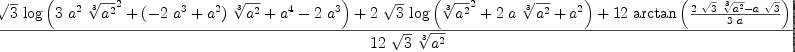(1)
Type: Union(f1: OrderedCompletion?(Expression(Integer)),...)

And here is a REDUCE command:

  \begin{reduce}
int(1/(a+z^3), z,0,1);
\end{reduce}


 load_package sfgamma; load_package defint; *** gamma declared operator int(1/(a+z^3), z,0,1); reduce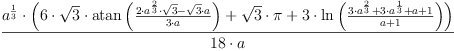## Common Mistakes

Please review the list of Common Mistakes and the list of MathAction Problems if you are have never used MathAction before. If you are learning to use Axiom and think that someone must have solved some particular problem before you, check this list of Common [Axiom Problems]?.

fricas
solve(a*x+b,x)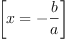(2)
Type: List(Equation(Fraction(Polynomial(Integer))))

exploring --Bill Page, Thu, 24 Apr 2008 07:00:25 -0700 reply
SandBoxNonAssociativeAlgebra

lexical scope --Bill Page, Sun, 11 May 2008 06:43:30 -0700 reply
Testing lexical scoping rules in SandBoxLexicalScope.

Combinatorial Sum --Bill Page, Fri, 16 May 2008 13:38:20 -0700 reply
SandBoxSum (like Product)

Symbolic computations --Bill Page, Thu, 22 May 2008 12:58:29 -0700 reply
SandBoxSymbolic

add inheritance issue --Bill Page, Sun, 25 May 2008 11:26:13 -0700 reply
For example: SandBoxLeftFreeModule

Added Preview and Cancel to comment form --page, Tue, 27 May 2008 15:08:43 -0700 reply
This is a test of the Preview and Cancel buttons:
fricas
integrate(sin x, x)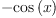(3)
Type: Union(Expression(Integer),...)

software archeology discovers another --Bill Page, Fri, 30 May 2008 21:01:37 -0700 reply
SandBoxSubsetCategory?

try iso-experiment/combinat --Bill Page, Tue, 03 Jun 2008 13:24:13 -0700 reply
SandBoxCombinat

Equation, Inequation, and Inequality --Bill Page, Mon, 09 Jun 2008 18:28:56 -0700 reply
SandBoxEquation? SandBoxInequation SandBoxInequality?

test aldor code --Bill Page, Wed, 18 Jun 2008 03:43:05 -0700 reply

gnuplottex --Bill Page, Tue, 24 Jun 2008 22:42:51 -0700 reply
SandBoxGnuPlotTex

implementing Integer from Cardinal (unsigned) numbers --Bill Page, Mon, 21 Jul 2008 06:34:23 -0700 reply
SandBoxDefineInteger

Attributes and categories --Bill Page, Fri, 25 Jul 2008 13:36:54 -0700 reply
SandBoxCommutativeCategory

Literal and Symbol in SPAD --Bill Page, Sun, 27 Jul 2008 02:09:57 -0700 reply
SandBoxLiteral

a category of partially ordered sets --Bill Page, Wed, 06 Aug 2008 17:26:50 -0700 reply
SandBoxPartiallyOrderedSet

in response to an exchange of emails with Gabriel Dos Reis concerning the validity of automatic translations of x >= y into not x < y, etc.

Document function selection process in the interpreter --Bill Page, Wed, 13 Aug 2008 18:10:26 -0700 reply
SandBox/interp/i-funsel.boot

Tensor Product of Polynomials --Bill Page, Sun, 24 Aug 2008 06:36:20 -0700 reply
SandBoxTensorProductPolynomial

Reflection --Bill Page, Mon, 08 Sep 2008 06:09:27 -0700 reply
)abbrev package REFL Reflect
Reflect(T:Type): with
constructor? : Symbol -> Boolean
constructor?(p:Symbol):Boolean == car(devaluate(T)$Lisp)$SExpression = convert(p)$SExpression spad  Compiling FriCAS source code from file /var/lib/zope2.10/instance/axiom-wiki/var/LatexWiki/2684009892188271010-25px004.spad using old system compiler. REFL abbreviates package Reflect ------------------------------------------------------------------------ initializing NRLIB REFL for Reflect compiling into NRLIB REFL compiling exported constructor? : Symbol -> Boolean Time: 0 SEC. (time taken in buildFunctor: 10) ;;; *** |Reflect| REDEFINED ;;; *** |Reflect| REDEFINED Time: 0.01 SEC. Cumulative Statistics for Constructor Reflect Time: 0.01 seconds finalizing NRLIB REFL Processing Reflect for Browser database: --->-->Reflect(constructor): Not documented!!!! --->-->Reflect((constructor? ((Boolean) (Symbol)))): Not documented!!!! --->-->Reflect(): Missing Description ; compiling file "/var/aw/var/LatexWiki/REFL.NRLIB/REFL.lsp" (written 18 FEB 2023 03:59:16 PM): ; /var/aw/var/LatexWiki/REFL.NRLIB/REFL.fasl written ; compilation finished in 0:00:00.011 ------------------------------------------------------------------------ Reflect is now explicitly exposed in frame initial Reflect will be automatically loaded when needed from /var/aw/var/LatexWiki/REFL.NRLIB/REFL fricas T1:=Integer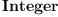(4) Type: Type fricas T2:=Polynomial Fraction T1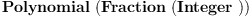(5) Type: Type fricas T3:=Complex T2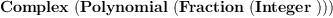(6) Type: Type fricas constructor?('Polynomial)$Reflect(T1)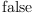(7)
Type: Boolean
fricas
constructor?('Polynomial)$Reflect(T2)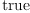(8) Type: Boolean fricas constructor?('Polynomial)$Reflect(T3)(9)
Type: Boolean
fricas
constructor?('Complex)\$Reflect(T3)(10)
Type: Boolean

Francois Maltey --Bill Page, Tue, 18 Nov 2008 19:11:35 -0800 reply
SandBoxConditionalFunctions

NonZeroInteger? --Bill Page, Tue, 02 Dec 2008 21:35:49 -0800 reply
SandBoxNonZeroInteger is an attempt to define the domain of Integers without 0.

Martin's "generator" mini-tutorial --Bill Page, Sat, 28 Feb 2009 09:00:14 -0800 reply
SandboxDelay

prototype for tensor products --Bill Page, Tue, 12 May 2009 12:49:48 -0700 reply
SandBoxTensorProduct by Franz Lehner

Riemann Surface --Bill Page, Sat, 20 Jun 2009 13:15:37 -0700 reply
SandBoxComplexManifold

Differential Algebra --Bill Page, Wed, 29 Jul 2009 09:07:26 -0700 reply
SandBoxDifferentialPolynomial

Grassmann Algebra --Bill Page, Thu, 10 Sep 2009 09:05:28 -0700 reply
SandBoxGrassmannIsometry - All mappings that preserve a given metric are given in terms of the decomposition of a general multivector.

Free Product --Bill Page, Fri, 18 Sep 2009 03:20:41 -0700 reply
SandBoxFreeProduct

This domain implements the free product of monoids (or groups) It is the coproduct in the category of monoids (groups). FreeProduct(A,B) is the monoid (group) whose elements are the reduced words in A and B, under the operation of concatenation followed by reduction:

• Remove identity elements (of either A or B)
• Replace a1a2 by its product in A and b1b2 by its product in B

FunctionWithCache? --Bill Page, Tue, 27 Oct 2009 14:51:49 -0700 reply
Franz Lehner provided the following example of caching the output of a function: SandBoxRemember

Hash Functions --Bill Page, Wed, 04 Nov 2009 00:11:08 -0800 reply
MortonCode (also called z-order) is a method of combining multidimensional "coordinates" into a one-dimensional coordinate or "code" that attempts to preserve locality, i.e. minimize the average Euclidean distance between coordinate locations associated with adjacent codes. Morton codes are computationally less expensive to convert to and from coordinate values than Hilbert codes.

Groebner Basis and Polynomial Ideals --Bill Page, Tue, 08 Feb 2011 14:48:48 -0800 reply
SandBoxGroebnerBasis examples from Ideals, Varieties, and Algorithms Third Edition, 2007

Frobenius Algebra --Bill Page, Fri, 11 Feb 2011 17:12:43 -0800 reply
FrobeniusAlgebraVectorSpacesAndPolynomialIdeals Classifying low dimensional Frobenius algebras

Compiling SPAD code from a string --Bill Page, Mon, 07 Mar 2011 15:59:46 -0800 reply

Sandbox with some simple Algebra [SimplifyingAlgebraicExpressions]?

Observer algebra --Bill Page, Thu, 26 Apr 2012 14:44:52 -0700 reply
SandBoxObserverAsIdempotent

Mathematica or sympy --Bill Page, Sat, 20 Apr 2013 01:35:47 +0000 reply
FriCAS
fricas
g:=1/(x*(1-a*(1-x)))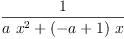(11)
Type: Fraction(Polynomial(Integer))
fricas
integrate(g,x)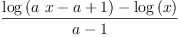(12)
Type: Union(Expression(Integer),...)

Sympy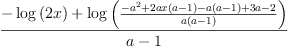(13)

Mathematica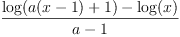(14)

Thomas Baruchel --Bill page, Sat, 22 Nov 2014 14:30:34 +0000 reply
fricas
)set output algebra on

fricas
)set output tex off
r1:=(16*x^14-125*x^10+150*x^6+375*x^2)/(256*x^16+480*x^12+1025*x^8+750*x^4 +625)
14        10        6        2
16 x   - 125 x   + 150 x  + 375 x
(13)  ------------------------------------------
16        12         8        4
256 x   + 480 x   + 1025 x  + 750 x  + 625
Type: Fraction(Polynomial(Integer))
fricas
r2:=integrate(r1,x=0..1);
Type: Union(f1: OrderedCompletion?(Expression(Integer)),...)
fricas
numeric r2
(15)  0.1239829519_8500639758
Type: Float
fricas
unparse(simplify(r2)::InputForm)
(16)
"(37268*5^(1/2)*62^(1/2)*((13144*5^(1/2)+(-30752))/(13144*5^(1/2)+(-29421)))^
(1/2)*atan(((1155*5^(1/2)+2200)*62^(1/2))/((106*5^(1/2)+248)*55^(1/2)*15125^(
1/4)*((13144*5^(1/2)+30752)/(13144*5^(1/2)+29421))^(1/2)*((((-1093842200)*5^(
1/2)+(-2445921555))*62^(1/2)*15125^(1/4)*((13144*5^(1/2)+30752)/(13144*5^(1/2
)+29421))^(1/2)+((790913912*5^(1/2)+1768578272)*15125^(1/2)+(108750662900*5^(
1/2)+243179512400)))/((790913912*5^(1/2)+1768578272)*15125^(1/2)))^(1/2)+((10
6*5^(1/2)+248)*55^(1/2)*15125^(1/4)*((13144*5^(1/2)+30752)/(13144*5^(1/2)+294
21))^(1/2)+((-55)*5^(1/2)+(-220))*55^(1/2)*62^(1/2))))+((823788*5^(1/2)+18401
60)*62^(1/2)*((13144*5^(1/2)+30752)/(13144*5^(1/2)+29421))^(1/2)*atan(((1155*
5^(1/2)+(-2200))*62^(1/2))/((106*5^(1/2)+(-248))*55^(1/2)*15125^(1/4)*((13144
*5^(1/2)+(-30752))/(13144*5^(1/2)+(-29421)))^(1/2)*((((-1093842200)*5^(1/2)+2
445921555)*62^(1/2)*15125^(1/4)*((13144*5^(1/2)+(-30752))/(13144*5^(1/2)+(-29
421)))^(1/2)+((790913912*5^(1/2)+(-1768578272))*15125^(1/2)+(108750662900*5^(
1/2)+(-243179512400))))/((790913912*5^(1/2)+(-1768578272))*15125^(1/2)))^(1/2
)+((106*5^(1/2)+(-248))*55^(1/2)*15125^(1/4)*((13144*5^(1/2)+(-30752))/(13144
*5^(1/2)+(-29421)))^(1/2)+((-55)*5^(1/2)+220)*55^(1/2)*62^(1/2))))+((823788*5
^(1/2)+1840160)*62^(1/2)*((13144*5^(1/2)+30752)/(13144*5^(1/2)+29421))^(1/2)*
atan(((1155*5^(1/2)+(-2200))*62^(1/2))/((106*5^(1/2)+(-248))*55^(1/2)*15125^(
1/4)*((13144*5^(1/2)+(-30752))/(13144*5^(1/2)+(-29421)))^(1/2)*(((1093842200*
5^(1/2)+(-2445921555))*62^(1/2)*15125^(1/4)*((13144*5^(1/2)+(-30752))/(13144*
5^(1/2)+(-29421)))^(1/2)+((790913912*5^(1/2)+(-1768578272))*15125^(1/2)+(1087
50662900*5^(1/2)+(-243179512400))))/((790913912*5^(1/2)+(-1768578272))*15125^
(1/2)))^(1/2)+((106*5^(1/2)+(-248))*55^(1/2)*15125^(1/4)*((13144*5^(1/2)+(-30
752))/(13144*5^(1/2)+(-29421)))^(1/2)+(55*5^(1/2)+(-220))*55^(1/2)*62^(1/2)))
)+(37268*5^(1/2)*62^(1/2)*((13144*5^(1/2)+(-30752))/(13144*5^(1/2)+(-29421)))
^(1/2)*atan(((1155*5^(1/2)+2200)*62^(1/2))/((106*5^(1/2)+248)*55^(1/2)*15125^
(1/4)*((13144*5^(1/2)+30752)/(13144*5^(1/2)+29421))^(1/2)*(((1093842200*5^(1/
2)+2445921555)*62^(1/2)*15125^(1/4)*((13144*5^(1/2)+30752)/(13144*5^(1/2)+294
21))^(1/2)+((790913912*5^(1/2)+1768578272)*15125^(1/2)+(108750662900*5^(1/2)+
243179512400)))/((790913912*5^(1/2)+1768578272)*15125^(1/2)))^(1/2)+((106*5^(
1/2)+248)*55^(1/2)*15125^(1/4)*((13144*5^(1/2)+30752)/(13144*5^(1/2)+29421))^
(1/2)+(55*5^(1/2)+220)*55^(1/2)*62^(1/2))))+(((-4081)*5^(1/2)+(-9548))*55^(1/
2)*62^(1/2)*((13144*5^(1/2)+(-30752))/(13144*5^(1/2)+(-29421)))^(1/2)*log((10
93842200*5^(1/2)+2445921555)*62^(1/2)*15125^(1/4)*((13144*5^(1/2)+30752)/(131
44*5^(1/2)+29421))^(1/2)+((790913912*5^(1/2)+1768578272)*15125^(1/2)+(1087506
62900*5^(1/2)+243179512400)))+(((-4081)*5^(1/2)+(-9548))*55^(1/2)*62^(1/2)*((
13144*5^(1/2)+30752)/(13144*5^(1/2)+29421))^(1/2)*log((1093842200*5^(1/2)+(-2
445921555))*62^(1/2)*15125^(1/4)*((13144*5^(1/2)+(-30752))/(13144*5^(1/2)+(-2
9421)))^(1/2)+(((-790913912)*5^(1/2)+1768578272)*15125^(1/2)+((-108750662900)
*5^(1/2)+243179512400)))+((4081*5^(1/2)+9548)*55^(1/2)*62^(1/2)*((13144*5^(1/
2)+30752)/(13144*5^(1/2)+29421))^(1/2)*log(((-1093842200)*5^(1/2)+2445921555)
*62^(1/2)*15125^(1/4)*((13144*5^(1/2)+(-30752))/(13144*5^(1/2)+(-29421)))^(1/
2)+(((-790913912)*5^(1/2)+1768578272)*15125^(1/2)+((-108750662900)*5^(1/2)+24
3179512400)))+((4081*5^(1/2)+9548)*55^(1/2)*62^(1/2)*((13144*5^(1/2)+(-30752)
)/(13144*5^(1/2)+(-29421)))^(1/2)*log(((-1093842200)*5^(1/2)+(-2445921555))*6
2^(1/2)*15125^(1/4)*((13144*5^(1/2)+30752)/(13144*5^(1/2)+29421))^(1/2)+((790
913912*5^(1/2)+1768578272)*15125^(1/2)+(108750662900*5^(1/2)+243179512400)))+
(((-823788)*5^(1/2)+(-1840160))*62^(1/2)*((13144*5^(1/2)+30752)/(13144*5^(1/2
)+29421))^(1/2)*atan(((231*5^(1/2)+(-440))*62^(1/2))/((106*5^(1/2)+(-248))*55
^(1/2)*15125^(1/4)*(11/(2*15125^(1/2)))^(1/2)*((13144*5^(1/2)+(-30752))/(1314
4*5^(1/2)+(-29421)))^(1/2)+((-11)*5^(1/2)+44)*55^(1/2)*62^(1/2)))+(((-823788)
*5^(1/2)+(-1840160))*62^(1/2)*((13144*5^(1/2)+30752)/(13144*5^(1/2)+29421))^(
1/2)*atan(((231*5^(1/2)+(-440))*62^(1/2))/((106*5^(1/2)+(-248))*55^(1/2)*1512
5^(1/4)*(11/(2*15125^(1/2)))^(1/2)*((13144*5^(1/2)+(-30752))/(13144*5^(1/2)+(
-29421)))^(1/2)+(11*5^(1/2)+(-44))*55^(1/2)*62^(1/2)))+((-37268)*5^(1/2)*62^(
1/2)*((13144*5^(1/2)+(-30752))/(13144*5^(1/2)+(-29421)))^(1/2)*atan(((231*5^(
1/2)+440)*62^(1/2))/((106*5^(1/2)+248)*55^(1/2)*15125^(1/4)*(11/(2*15125^(1/2
)))^(1/2)*((13144*5^(1/2)+30752)/(13144*5^(1/2)+29421))^(1/2)+((-11)*5^(1/2)+
(-44))*55^(1/2)*62^(1/2)))+((-37268)*5^(1/2)*62^(1/2)*((13144*5^(1/2)+(-30752
))/(13144*5^(1/2)+(-29421)))^(1/2)*atan(((231*5^(1/2)+440)*62^(1/2))/((106*5^
(1/2)+248)*55^(1/2)*15125^(1/4)*(11/(2*15125^(1/2)))^(1/2)*((13144*5^(1/2)+30
752)/(13144*5^(1/2)+29421))^(1/2)+(11*5^(1/2)+44)*55^(1/2)*62^(1/2)))+(5830*5
^(1/2)+13640)*55^(1/2)*15125^(1/4)*((13144*5^(1/2)+(-30752))/(13144*5^(1/2)+(
-29421)))^(1/2)*((13144*5^(1/2)+30752)/(13144*5^(1/2)+29421))^(1/2)))))))))))
))/((89782*5^(1/2)+210056)*55^(1/2)*15125^(1/4)*((13144*5^(1/2)+(-30752))/(13
144*5^(1/2)+(-29421)))^(1/2)*((13144*5^(1/2)+30752)/(13144*5^(1/2)+29421))^(1
/2))"
Type: String
fricas
r3:=integrate(r1,x);
Type: Union(Expression(Integer),...)
fricas
unparse(simplify(r3)::InputForm)
(18)
"((85184*x^8+79860*x^4+133100)*5^(1/2)*62^(1/2)*((13144*5^(1/2)+(-30752))/(13
144*5^(1/2)+(-29421)))^(1/2)*atan(((1155*5^(1/2)+2200)*62^(1/2))/((106*5^(1/2
)+248)*55^(1/2)*15125^(1/4)*((13144*5^(1/2)+30752)/(13144*5^(1/2)+29421))^(1/
2)*((((-1093842200)*x*5^(1/2)+(-2445921555)*x)*62^(1/2)*15125^(1/4)*((13144*5
^(1/2)+30752)/(13144*5^(1/2)+29421))^(1/2)+((790913912*x^2*5^(1/2)+1768578272
*x^2)*15125^(1/2)+(108750662900*5^(1/2)+243179512400)))/((790913912*5^(1/2)+1
768578272)*15125^(1/2)))^(1/2)+((106*x*5^(1/2)+248*x)*55^(1/2)*15125^(1/4)*((
13144*5^(1/2)+30752)/(13144*5^(1/2)+29421))^(1/2)+((-55)*5^(1/2)+(-220))*55^(
1/2)*62^(1/2))))+(((1882944*x^8+1765260*x^4+2942100)*5^(1/2)+(4206080*x^8+394
3200*x^4+6572000))*62^(1/2)*((13144*5^(1/2)+30752)/(13144*5^(1/2)+29421))^(1/
2)*atan(((1155*5^(1/2)+(-2200))*62^(1/2))/((106*5^(1/2)+(-248))*55^(1/2)*1512
5^(1/4)*((13144*5^(1/2)+(-30752))/(13144*5^(1/2)+(-29421)))^(1/2)*((((-109384
2200)*x*5^(1/2)+2445921555*x)*62^(1/2)*15125^(1/4)*((13144*5^(1/2)+(-30752))/
(13144*5^(1/2)+(-29421)))^(1/2)+((790913912*x^2*5^(1/2)+(-1768578272)*x^2)*15
125^(1/2)+(108750662900*5^(1/2)+(-243179512400))))/((790913912*5^(1/2)+(-1768
578272))*15125^(1/2)))^(1/2)+((106*x*5^(1/2)+(-248)*x)*55^(1/2)*15125^(1/4)*(
(13144*5^(1/2)+(-30752))/(13144*5^(1/2)+(-29421)))^(1/2)+((-55)*5^(1/2)+220)*
55^(1/2)*62^(1/2))))+(((1882944*x^8+1765260*x^4+2942100)*5^(1/2)+(4206080*x^8
+3943200*x^4+6572000))*62^(1/2)*((13144*5^(1/2)+30752)/(13144*5^(1/2)+29421))
^(1/2)*atan(((1155*5^(1/2)+(-2200))*62^(1/2))/((106*5^(1/2)+(-248))*55^(1/2)*
15125^(1/4)*((13144*5^(1/2)+(-30752))/(13144*5^(1/2)+(-29421)))^(1/2)*(((1093
842200*x*5^(1/2)+(-2445921555)*x)*62^(1/2)*15125^(1/4)*((13144*5^(1/2)+(-3075
2))/(13144*5^(1/2)+(-29421)))^(1/2)+((790913912*x^2*5^(1/2)+(-1768578272)*x^2
)*15125^(1/2)+(108750662900*5^(1/2)+(-243179512400))))/((790913912*5^(1/2)+(-
1768578272))*15125^(1/2)))^(1/2)+((106*x*5^(1/2)+(-248)*x)*55^(1/2)*15125^(1/
4)*((13144*5^(1/2)+(-30752))/(13144*5^(1/2)+(-29421)))^(1/2)+(55*5^(1/2)+(-22
0))*55^(1/2)*62^(1/2))))+((85184*x^8+79860*x^4+133100)*5^(1/2)*62^(1/2)*((131
44*5^(1/2)+(-30752))/(13144*5^(1/2)+(-29421)))^(1/2)*atan(((1155*5^(1/2)+2200
)*62^(1/2))/((106*5^(1/2)+248)*55^(1/2)*15125^(1/4)*((13144*5^(1/2)+30752)/(1
3144*5^(1/2)+29421))^(1/2)*(((1093842200*x*5^(1/2)+2445921555*x)*62^(1/2)*151
25^(1/4)*((13144*5^(1/2)+30752)/(13144*5^(1/2)+29421))^(1/2)+((790913912*x^2*
5^(1/2)+1768578272*x^2)*15125^(1/2)+(108750662900*5^(1/2)+243179512400)))/((7
90913912*5^(1/2)+1768578272)*15125^(1/2)))^(1/2)+((106*x*5^(1/2)+248*x)*55^(1
/2)*15125^(1/4)*((13144*5^(1/2)+30752)/(13144*5^(1/2)+29421))^(1/2)+(55*5^(1/
2)+220)*55^(1/2)*62^(1/2))))+((((-9328)*x^8+(-8745)*x^4+(-14575))*5^(1/2)+((-
21824)*x^8+(-20460)*x^4+(-34100)))*55^(1/2)*62^(1/2)*((13144*5^(1/2)+(-30752)
)/(13144*5^(1/2)+(-29421)))^(1/2)*log((1093842200*x*5^(1/2)+2445921555*x)*62^
(1/2)*15125^(1/4)*((13144*5^(1/2)+30752)/(13144*5^(1/2)+29421))^(1/2)+((79091
3912*x^2*5^(1/2)+1768578272*x^2)*15125^(1/2)+(108750662900*5^(1/2)+2431795124
00)))+(((9328*x^8+8745*x^4+14575)*5^(1/2)+(21824*x^8+20460*x^4+34100))*55^(1/
2)*62^(1/2)*((13144*5^(1/2)+30752)/(13144*5^(1/2)+29421))^(1/2)*log((10938422
00*x*5^(1/2)+(-2445921555)*x)*62^(1/2)*15125^(1/4)*((13144*5^(1/2)+(-30752))/
(13144*5^(1/2)+(-29421)))^(1/2)+((790913912*x^2*5^(1/2)+(-1768578272)*x^2)*15
125^(1/2)+(108750662900*5^(1/2)+(-243179512400))))+((((-9328)*x^8+(-8745)*x^4
+(-14575))*5^(1/2)+((-21824)*x^8+(-20460)*x^4+(-34100)))*55^(1/2)*62^(1/2)*((
13144*5^(1/2)+30752)/(13144*5^(1/2)+29421))^(1/2)*log(((-1093842200)*x*5^(1/2
)+2445921555*x)*62^(1/2)*15125^(1/4)*((13144*5^(1/2)+(-30752))/(13144*5^(1/2)
+(-29421)))^(1/2)+((790913912*x^2*5^(1/2)+(-1768578272)*x^2)*15125^(1/2)+(108
750662900*5^(1/2)+(-243179512400))))+(((9328*x^8+8745*x^4+14575)*5^(1/2)+(218
24*x^8+20460*x^4+34100))*55^(1/2)*62^(1/2)*((13144*5^(1/2)+(-30752))/(13144*5
^(1/2)+(-29421)))^(1/2)*log(((-1093842200)*x*5^(1/2)+(-2445921555)*x)*62^(1/2
)*15125^(1/4)*((13144*5^(1/2)+30752)/(13144*5^(1/2)+29421))^(1/2)+((790913912
*x^2*5^(1/2)+1768578272*x^2)*15125^(1/2)+(108750662900*5^(1/2)+243179512400))
)+((5830*x^7+40810*x^3)*5^(1/2)+(13640*x^7+95480*x^3))*55^(1/2)*15125^(1/4)*(
(13144*5^(1/2)+(-30752))/(13144*5^(1/2)+(-29421)))^(1/2)*((13144*5^(1/2)+3075
2)/(13144*5^(1/2)+29421))^(1/2)))))))))/(((205216*x^8+192390*x^4+320650)*5^(1
/2)+(480128*x^8+450120*x^4+750200))*55^(1/2)*15125^(1/4)*((13144*5^(1/2)+(-30
752))/(13144*5^(1/2)+(-29421)))^(1/2)*((13144*5^(1/2)+30752)/(13144*5^(1/2)+2
9421))^(1/2))"
Type: String
fricas
r4:=D(r3,x);
Type: Expression(Integer)
fricas
--simplify(r1-r4)
--normalize(r1-r4)
r5:=eval(r1-r4,x=10);
Type: Expression(Integer)
fricas
numeric r5
(21)  - 0.8167478186_7186562196 E -7
Type: Float

LaTeX output formatting --Bill page, Mon, 29 Dec 2014 19:38:46 +0000 reply
fricas
)set output algebra on
sin(x^b)
b
(22)  sin(x )
Type: Expression(Integer)
fricas
D(%,x)
b - 1     b
(23)  b x     cos(x )
Type: Expression(Integer)
fricas
D(%,x)
2  b - 1 2     b      2      b - 2     b
(24)  - b (x     ) sin(x ) + (b  - b)x     cos(x )
Type: Expression(Integer)
fricas
)set output algebra off

fricas
)set output tex on

fricas
guessRec([1,1,0,1,- 1,2,- 1,5,- 4,29,- 13,854,- 685])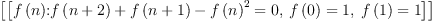(15)
Type: List(Expression(Integer))fricas
integral(x^2, x)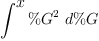(16)
Type: Expression(Integer)
fricas
integrate(x^2, x)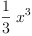(17)
Type: Polynomial(Fraction(Integer))

fricas
integrate(log(m*x+b),x)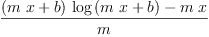(18)
Type: Union(Expression(Integer),...)

fricas
integrate(log(m*x+b) * exp(-(log(x) - mu)^2 / (2*sigma^2)) / (X*sigma*sqrt(2*pi)), x)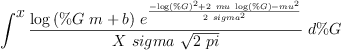(19)
Type: Union(Expression(Integer),...)

fricas
integrate(log(b) * exp(-(log(x) - mu)^2 / (2*sigma^2)) / (X*sigma*sqrt(2*pi)), x)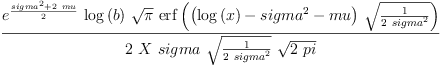(20)
Type: Union(Expression(Integer),...)

 Subject: (replying)   Be Bold !! ( 17 subscribers )